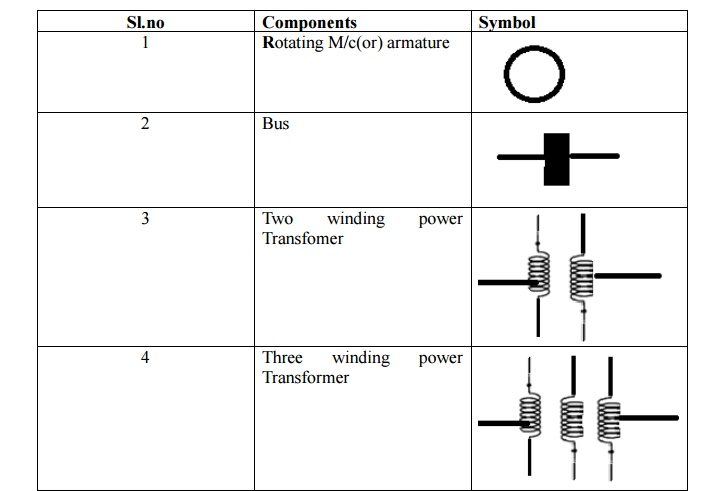Home | | Power System Analysis | Single Line diagram of an Electrical system

# Single Line diagram of an Electrical system

In general electrical power systems are represented by a one line diagram (or) single line diagram

SINGLE LINE DIAGRAM

## Single line diagram

In general electrical power systems are represented by a one line diagram (or) single line diagram

A single line diagram of a power system shows the main connections & arrangements of components in a simplified manner

Pictorial representation of the entire power system from generating end to the consumer premises is known as single line diagram

## Standard symbolsSingle Line diagram of an Electrical systemØ  One line diagram of a very simple power system

Ø  Two generators one grounded through a reactor and one through a resister connected to a bus and through a step up transformer to a transmission lines

Ø  Another generator grounded a reactor is connected a bus and through a transformer to the opposite end of the transmission line

Ø  A load is connected to each bus

Ø  On the diagram information about the loads the ratings of the generators and transformers and reactance of different components of the circuit is often given

Ø  It is important to know the location of points where a system is connected to ground to calculate the amount of current flowing when an unsymmetrical fault involving ground occur

## Equivalent circuit for various power system components:

(i). Generators(ii). Transmission lines(iii). Transformer